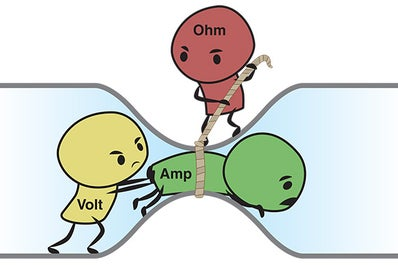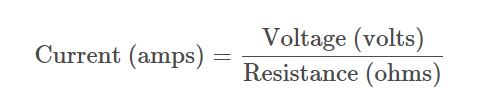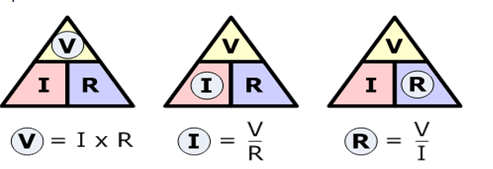# Ohm’s Law Study Guide

INTRODUCTION

The modern world is entirely dominated by electricity and our ability to control it. How often have you stopped to think about all the things you take for granted- such as the light in your house, the air conditioner, the water heater, the toaster, and electronic gadgets?None of this would function without Ohm’s law! This fundamental principle discovered about two hundred years ago made it possible to create machines that built the world we know today. So what does Ohm’s law say? Let’s find out!Source

### WHAT IS OHM’S LAW?Source

When learning about electrical circuits, there are three important parameters you need to know:

Voltage (v): Voltage measures the electrical potential difference across two points. Think of it as a pressure source in an electrical circuit that pushes electrons (current) through the wires. The flow of electrons gets work done, such as illuminating a bulb or running a motor.

Current (I): Current measures how many electrons flow across a given point under unit time. Think of current as the number of electrons flowing in the wires. The unit of current is ampere (A) or amps. 1 ampere of current equals 1 coulomb (6.24 x 1018) electrons flowing past a point in 1 second. It is like measuring how much water flows out of a garden hose in under 1 second.

Resistance (R): Simply put, resistance is how much a wire or conductor resists the current flow in an electrical circuit. Copper has a low resistance; hence it is used as a conductor, whereas rubber has such a high resistance that it completely restricts the flow of current. Different materials have different levels of resistance to the flow of current.
Define Ohm’s Law: This law states that the current flowing in a conductor is directly proportional to the voltage across the conductor. Mathematically the ohm’s law equation states that: V ∝ I Or V=RI

R is the constant of proportionality which is resistance. The value of R is different for different conductors.Source

Ohm’s law draws a relationship between voltage, current, and resistance. The ohm’s law formulas can be used to deduce the current flowing in a conductor or the resistance or the voltage if any of the two values is known.

### SOME PRACTICAL OHM LAW EXAMPLES:

• Ohm’s law is the principle behind the working of safety fuses and circuit breakers. Fuses are rated for a fixed current and melt when more current flows through.
• Electronic device design.
• Speed control of fans using a potentiometer.
• Functioning of heating elements.

## CONCLUSION:

• Ohm’s law gives a conductor the relationship between voltage, current, and resistance.
• Voltage measures the electrical potential difference across two points in a conductor.
• Current measures how many electrons flow across a given point under unit time.
• Resistance is how much a wire or conductor resists the current flow in an electrical circuit.

### FAQs:

1. What is Ohm’s law explain?

Ohm’s law gives the relationship between voltage, current, and resistance in a conductor. To state ohms law, the current across two points in a conductor is directly proportional to the voltage across the points.

2. What is Ohm’s law? Write the formula?

The current flowing in a conductor is directly proportional to the voltage across the conductor.

Mathematically: V=RI

3. What are the three formulas in Ohm’s law?

V=RI, I=V/R, and R=V/I

We hope you enjoyed studying this lesson and learned something cool about Ohm’s Law! Join our Discord community to get any questions you may have answered and to engage with other students just like you! Don’t forget to download our App to experience our fun, VR classrooms – we promise, it makes studying much more fun! 😎

## SOURCES:

1. 20.10 Ohm’s Law. https://flexbooks.ck12.org/cbook/ck-12-middle-school-physical-science-flexbook-2.0/section/20.10/primary/lesson/ohms-law-ms-ps/. Accessed 28 Jan 2022.
2. What is voltage?. https://www.fluke.com/en-in/learn/blog/electrical/what-is-voltage#:~:text=Voltage%20is%20the%20pressure%20from,measured%20in%20volts%20(V). Accessed 28 Jan 2022.
3. 20.5 Current. https://flexbooks.ck12.org/cbook/ck-12-middle-school-physical-science-flexbook-2.0/section/20.5/primary/lesson/electric-current-ms-ps/https://www.fluke.com/en-in/learn/blog/electrical/what-is-resistance. Accessed 28 Jan 2022.
]]>